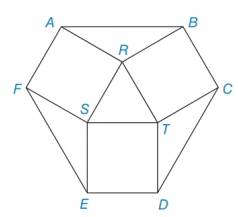Chapter 8.3, Problem 41EElementary Geometry For College St...

7th Edition
Alexander + 2 others
ISBN: 9781337614085

Solutions

Chapter
SectionElementary Geometry For College St...

7th Edition
Alexander + 2 others
ISBN: 9781337614085
Textbook Problem

For equilateral Δ ARST, RT = 6. Squares and triangles on the sides of Δ RST determine hexagon ABCDEF. Find the area of ABCDEF.To determine

To find:

The area of the hexagon.

Explanation

Concept:

1) The area of the square is given by, A = s2 where s is side of the square.

2) The area of a triangle can be expressed using the lengths of two sides and the sine of the included angle, A=12×absinC

3) The area of the equilateral triangle is given by, A=34s2 where s is side of the equilateral triangle.

Calculation:

It is given that the side of the square is 6 units.

Use the formula of area of the square, A = s2

Substitute s = 6 in A = s2.

Asquare=(6)2=36units2

It is given that the side of the equilateral triangle is 6 units.

Use the formula of area of the equilateral triangle, A=34s2

Substitute s=6 in A=34s2.

ARST=34(6)2=34(36)=15.59units2

Still sussing out bartleby?

Check out a sample textbook solution.

See a sample solution

The Solution to Your Study Problems

Bartleby provides explanations to thousands of textbook problems written by our experts, many with advanced degrees!

Get Started

Let f be the function defined by f(x)={x2+1ifx0xifx0 Find f(2), f(0), and f(1).

Applied Calculus for the Managerial, Life, and Social Sciences: A Brief Approach

39. Find the average rate of change of

Mathematical Applications for the Management, Life, and Social Sciences

Subtract and check: 60,0009,876_

Elementary Technical Mathematics

True or False: If converges and converges, then converges.

Study Guide for Stewart's Multivariable Calculus, 8th

True or False: If f(x) = F(x), then baf(x)dx=F(b)F(a).

Study Guide for Stewart's Single Variable Calculus: Early Transcendentals, 8th